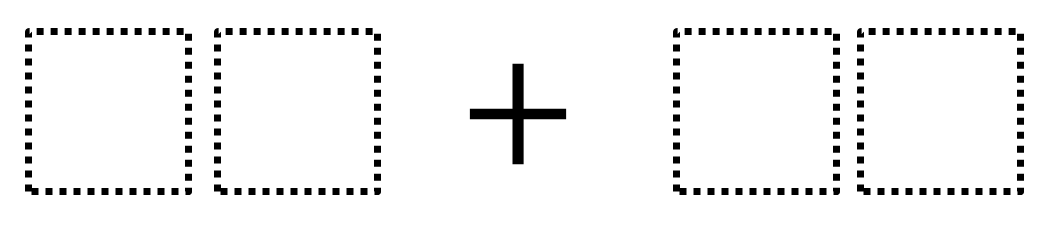Directions: Using the digits 1 to 9 at most one time each, place a digit in each box to make the smallest (or largest) sum.### Hint

In the two-digit number, what does the digit on the left represent?  What does the digit on the right represent?

97 + 86 is one answer for the largest sum.  13 + 24 is the smallest sum.

Source: Robert Kaplinsky

### Check Also

Directions: Use the digits 1-9, at most once, to complete the equation. What is the …

1.My second grade class loves coming up with variations on this. My personal favorite so far is what is the largest sum you can make with the biggest difference between the two numbers? I also like what is the largest/smallest you can make with only even or odd numbers?

2.ANTHONY FITZPATRICK

96+87 for largest and 14+23 for smallest . Since you are only moving a “one”, the value of the sum remains the same

3.40+60=100

4.97+86

5.98+76

6.lasr :13+24=37
high:97+86=183

7.8.9.19 + 43

10.Smallest :13+24=37
highest:97+86=183

11.12 + 34 =46

98 + 76 = 174

12.small 38+50 = 88
large 70+ 40 = 110

13.14.97+86
13+24

15.smallest-15+27
largest-45+36

16.16+82=98

17.12+36=48

no repeating numbers and all numbers are less than 9

18.19.Largest 97+86
Smallest 13+24

20.LARGE 87+96
SMALL 1+2

21.22.captain obvious jr.

hello i got the answer of 97+86 i got it cause im smart